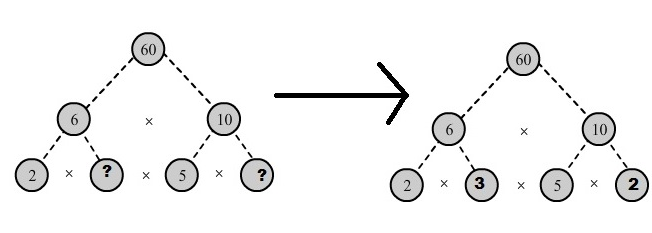×#### Thank you for registering.

One of our academic counsellors will contact you within 1 working day.

Click to Chat

1800-1023-196

+91-120-4616500

CART 0

• 0

MY CART (5)

Use Coupon: CART20 and get 20% off on all online Study Material

ITEM
DETAILS
MRP
DISCOUNT
FINAL PRICE
Total Price: Rs.

There are no items in this cart.
Continue Shopping• Complete JEE Main/Advanced Course and Test Series
• OFFERED PRICE: Rs. 15,900
• View Details

```Chapter 2: Playing With Numbers – Exercise 2.4

Question: 1

In which of the following expressions, prime factorization has been done?

Solution:

(i) 24 = 2 x 3 x 4 is not a prime factorization as 4 is not a prime number.

(ii) 56 = 1 x 7 x 2 x2 x 2 is not a prime factorization as 1 is not a prime number.

(iii) 70 = 2 x 5 x 7 is a prime factorization as 2, 5, and 7 are prime numbers.

(iv) 54 = 2 x 3 x 9 is not a prime factorization as 9 is not a prime number.

Question: 2

Determine prime factorization of each of the following numbers:

Solution:

(i) 216

We have:

2
216

2
108

2
54

3
27

3
9

3
3

1

Therefore, Prime factorization of 216 = 2 x 2 x 2 x 3 x 3

(ii) 420

We have:

2
420

2
210

3
105

5
35

7
7

1

Therefore, Prime factorization of 420 = 2 x 2 x 3 x 5 x 7

(iii) 468

We have:

2
468

2
234

3
117

3
39

13
13

1

Therefore, Prime factorization of 468 = 2 x 2 x 3 x 3 x 13

(iv) 945

We have:

3
945

3
315

3
105

5
35

7
7

1

Therefore, Prime factorization of 945 = 3 x 3 x 3 x 5 x 7

(v) 7325

We have:

5
7325

5
1465

293
293

1

Therefore, Prime factorization of 732 5= 5 x 5 x 293

(vi) 13915

We have:

5
13915

11
2783

11
253

23
23

1

Therefore, Prime factorization of 13915 = 5 x 11 x 11 x 23

Question: 3

Write the smallest 4-digit number and express it as a product of primes.

Solution:

The smallest 4-digit number is 1000.

1000 = 2 x 500

= 2 × 2 × 250

= 2 × 2 × 2 × 125

= 2 × 2 × 2 × 5 × 25

= 2 × 2 × 2 × 5 × 5 × 5

Therefore, 1000 = 2 × 2 × 2 × 5 × 5 × 5

Question: 4

Write the largest 4-digit number and express it as product of primes.

Solution:

The largest 4-digit number is 9999.

We have:

3
9999

3
3333

11
1111

101
101

1

Hence, the largest 4-digit number 9999 can be expressed in the form of its prime factors as 3 x 3 x 11 x 101.

Question: 5

Find the prime factors of 1729. Arrange the factors in ascending order, and find the relation between two consecutive prime factors.

Solution:

The given number is 1729.

We have:

7
1729

13
247

19
19

Thus, the number 1729 can be expressed in the form of its prime factors ass 7 x 13 x19.

Relation between its two consecutive prime factors:

The consecutive prime factors of the given number are 7, 13 and 19.

Clearly, 13 – 7 = 6 and 19 – 13 =6

Here, in two consecutive prime factors, the latter is 6 more than the previous one.

Question: 6

Which factors are not included in the prime factorization of a composite number?

Solution:

1 and the number itself are not included in the prime factorization of a composite number.

Example: 4 is a composite number.

Prime factorization of 4 = 2 x 2.

Question: 7

Here are two different factor trees for 60. Write the missing numbers:

Solution:

(i) Since 6 = 2 x 3 and 10= 5 x 2. We have:(ii) Since 60 = 30 x 2.

30= 10 x 3 and 10 = 5 x 2 we have:```### Course Features

• 728 Video Lectures
• Revision Notes
• Previous Year Papers
• Mind Map
• Study Planner
• NCERT Solutions
• Discussion Forum
• Test paper with Video Solution